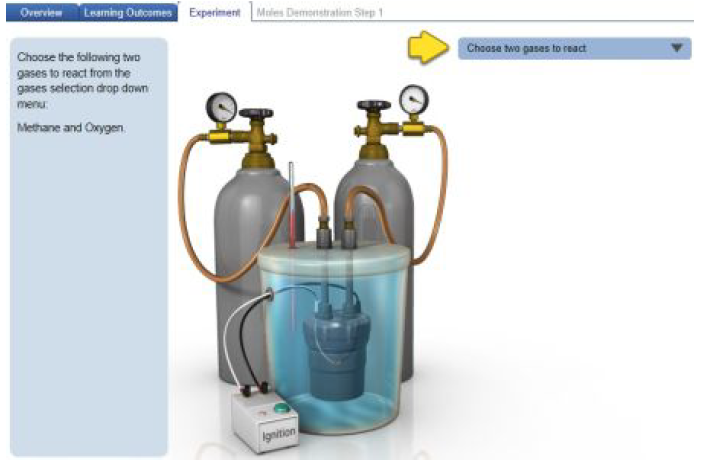# Problem: The balanced reaction for the combustion of ethane is shown in the table. You allow 2.5 mol of ethane (C2H6) to react with 3.6 mol oxygen (O2). Complete the following ICF table, which may be done using the Simulation, to indicate the amounts of all chemical species for the initial, change, and final conditions.Balanced chemical equations, which represent reactions, include coefficients that describe the ratio between the reactants and products. A balanced chemical equation not only describes how many molecules or moles react with each other, but it also embodies the fact that matter and mass are conserved in a reaction. This means that all of the atoms present among the reactants will be present in the same amount among the products. For example, the combustion reaction between methane and oxygen can be depicted in a balanced chemical equation:CH4(g) + 2O2(g) → CO2(g) + 2 H2O(g)Click on the image below to explore this simulation, which allows you run reactions between various gases and specify their amounts. When you click the simulation link, you will be able toselect among Overview, Learning Outcomes, and Experiment tabs.When the simulation is opened, you should see an overview describing how stoichiometry relates to the changes in the amounts of reactants and products in a reaction. Clicking the menu tabs toward the top of the screen can switch among an overview of stoichiometry, the learning outcomes of the simulation, and three experiments. Two of the experiments are guided tutorials that demonstrate how moles (Run Moles Demonstration) and both mass and moles (Run Grams Demonstration) change during a reaction. You can also run your own experiment by clicking Run Experiment. For any of the experiments, follow the step-by-step procedures that are explained on the left, and your interactions within the guided tutorial will be guided by an undulating yellow arrow.

###### FREE Expert Solution
88% (378 ratings)View Complete Written Solution
###### Problem Details

The balanced reaction for the combustion of ethane is shown in the table. You allow 2.5 mol of ethane (C2H6) to react with 3.6 mol oxygen (O2). Complete the following ICF table, which may be done using the Simulation, to indicate the amounts of all chemical species for the initial, change, and final conditions.

Balanced chemical equations, which represent reactions, include coefficients that describe the ratio between the reactants and products. A balanced chemical equation not only describes how many molecules or moles react with each other, but it also embodies the fact that matter and mass are conserved in a reaction. This means that all of the atoms present among the reactants will be present in the same amount among the products. For example, the combustion reaction between methane and oxygen can be depicted in a balanced chemical equation:

CH4(g) + 2O2(g) → CO2(g) + 2 H2O(g)

Click on the image below to explore this simulation, which allows you run reactions between various gases and specify their amounts. When you click the simulation link, you will be able to
select among Overview, Learning Outcomes, and Experiment tabs.When the simulation is opened, you should see an overview describing how stoichiometry relates to the changes in the amounts of reactants and products in a reaction. Clicking the menu tabs toward the top of the screen can switch among an overview of stoichiometry, the learning outcomes of the simulation, and three experiments.

Two of the experiments are guided tutorials that demonstrate how moles (Run Moles Demonstration) and both mass and moles (Run Grams Demonstration) change during a reaction. You can also run your own experiment by clicking Run Experiment. For any of the experiments, follow the step-by-step procedures that are explained on the left, and your interactions within the guided tutorial will be guided by an undulating yellow arrow.